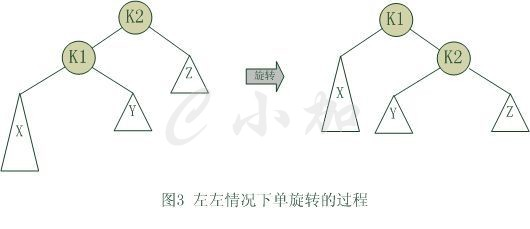/*--------------------CSS部分-------------------*/ /*--------------------JS部分-------------------*/

# 浅谈数据结构-平衡二叉树# 一、定义及原理距离插入结点最近的，且平衡因子的绝对值大于1的结点为根的子树，称为最小不平衡子树。如下图所示，当插入结点37时，距离它最近的平衡因子的绝对值超过1的结点是58。typedef struct BitNode
{
int data;
int bf;
struct BitNode *lchild, *rchild;
}BitNode, *BiTree;

# 二、结点插入

## 1、插入原理## 2.旋转问题1、6节点的左子树3节点高度比右子树7节点大2，左子树3节点的左子树1节点高度大于右子树4节点，这种情况成为左左。

2、6节点的左子树2节点高度比右子树7节点大2，左子树2节点的左子树1节点高度小于右子树4节点，这种情况成为左右。

3、2节点的左子树1节点高度比右子树5节点小2，右子树5节点的左子树3节点高度大于右子树6节点，这种情况成为右左。

4、2节点的左子树1节点高度比右子树4节点小2，右子树4节点的左子树3节点高度小于右子树6节点，这种情况成为右右。

## 3、旋转操作void R_rotate(BiTree *t)
{
BiTree s;
s = (*t)->lchild;                    //s指向t的左子树根结点
(*t)->lchild = s->rchild;          //s的右子树挂接为t的左子树
s->rchild = (*t);
*t = s;                                //t指向新的根结点
}

void L_rotate(BiTree *t)
{
BiTree s;
s = (*t)->rchild;                    //s指向t的右子树根结点
(*t)->rchild = s->lchild;          //s的左子树挂接为t的右子树
s->lchild = (*t);
*t = s;                                //t指向新的根结点
}为使树恢复平衡，我们需要进行两步，第一步，把k1作为根，进行一次z左旋转，旋转之后就变成了左左情况，所以第二步再进行一次右旋转，最后得到了一棵以k2为根的平衡二叉树树。

## 3、旋转代码分析

#define LH +1 /*  左高 */
#define EH 0  /*  等高 */
#define RH -1 /*  右高 */

/*  对以指针T所指结点为根的二叉树作左平衡旋转处理 */
/*  本算法结束时，指针T指向新的根结点 */
void LeftBalance(BiTree *T)
{
BiTree L,Lr;
L = (*T)->lchild;                                      /*  L指向T的左子树根结点 */
switch(L->bf)
{
/* 检查T的左子树的平衡度，并作相应平衡处理 */
case LH:                   /* 新结点插入在T的左孩子的左子树上，要作单右旋处理 */
(*T)->bf=L->bf=EH;
R_Rotate(T);
break;
case RH:                   /* 新结点插入在T的左孩子的右子树上，要作双旋处理 */
Lr=L->rchild;                    /* Lr指向T的左孩子的右子树根 */
switch(Lr->bf)
{   /* 修改T及其左孩子的平衡因子 */
case LH: (*T)->bf=RH;
L->bf=EH;
break;
case EH: (*T)->bf=L->bf=EH;
break;
case RH: (*T)->bf=EH;
L->bf=LH;
break;
}
Lr->bf=EH;
L_Rotate(&(*T)->lchild);         /* 对T的左子树作左旋平衡处理 */
R_Rotate(T);             /* 对T作右旋平衡处理 */
}
}

（1）函数被调用，传入一个需调整平衡型的子树T，根节点为k3,由于LeftBalance函数被调用时，其实是已经确认当前子树是不平衡的状态，且左子树的高度大于右子树的高度。换句话说，此时T的根结点应该是平衡因子BF的值大于1的数。k3的BF为2

（2）将T的左孩子赋值给L。L指向K1.

（3）然后是分支判断。

（4）当L（k1)的平衡因子为LH，即为1时，表明它与根结点的BF值符号相同，因此，将它们的BF值都改为0，并进行右旋（顺时针）操作，是左左情况

（5）当L的平衡因子为RH时，即为-1时，表明它与根结点的BF值符号相反，此时需要做双旋操作。针对L的右孩子k2的BF作判断，修改结点T(k3)和L(k1)的BF值。将当前的Lr的BF改为0。从图中看到K2的左结点是连接到K1的右子树上，右结点连接到K3的左子树

（6）对根结点的左子树进行左旋，以K1为根节点进行左旋转，形成左左情况。

（7）对根结点K3进行右旋，完成平衡操作。

<strong>#include <stdio.h>
#include <stdlib.h>

#define OK 1
#define ERROR 0
#define TRUE 1
#define FALSE 0
#define MAXSIZE 100                     /* 存储空间初始分配量 */

typedef int Status;                     /* Status是函数的类型,其值是函数结果状态代码，如OK等 */

/* 二叉树的二叉链表结点结构定义 */
typedef  struct BitNode                 /* 结点结构 */
{
int data;                           /* 结点数据 */
int bf;                             /*  结点的平衡因子 */
struct BitNode *lchild, *rchild;    /* 左右孩子指针 */
} BitNode, *BiTree;

/* 对以p为根的二叉排序树作右旋处理 */
/* 处理之后p指向新的树根结点，即旋转处理之前的左子树的根结点 */
//右旋-顺时针旋转(如LL型就得对根结点做该旋转)
void R_Rotate(BiTree *P)
{
BiTree L;
L=(*P)->lchild;                      /*  L指向P的左子树根结点 */
(*P)->lchild=L->rchild;               /*  L的右子树挂接为P的左子树 */
L->rchild=(*P);
*P=L;                               /*  P指向新的根结点 */
}

/* 对以P为根的二叉排序树作左旋处理， */
/* 处理之后P指向新的树根结点，即旋转处理之前的右子树的根结点0  */
//左旋-逆时针旋转(如RR型就得对根结点做该旋转)
void L_Rotate(BiTree *P)
{
BiTree R;
R = (*P)->rchild;                    /* R指向P的右子树根结点 */
(*P)->rchild = R->lchild;         /* R的左子树挂接为P的右子树 */
R->lchild = (*P);
*P = R;                             /* P指向新的根结点 */
}

#define LH +1                           /*  左高 */
#define EH 0                            /*  等高 */
#define RH -1                           /*  右高 */

/*  对以指针T所指结点为根的二叉树作左平衡旋转处理 */
/*  本算法结束时，指针T指向新的根结点 */
void LeftBalance(BiTree *T)
{
BiTree L,Lr;
L = (*T)->lchild;                    /*  L指向T的左子树根结点 */
switch(L->bf)
{
/* 检查T的左子树的平衡度，并作相应平衡处理 */
case LH:                        /* 新结点插入在T的左孩子的左子树上，要作单右旋处理 */
(*T)->bf=L->bf=EH;
R_Rotate(T);
break;
case RH:                        /* 新结点插入在T的左孩子的右子树上，要作双旋处理 */ //
Lr=L->rchild;                /* Lr指向T的左孩子的右子树根 */
switch(Lr->bf)
{
/* 修改T及其左孩子的平衡因子 */
case LH:
(*T)->bf=RH;
L->bf=EH;
break;
case EH:
(*T)->bf=L->bf=EH;
break;
case RH:
(*T)->bf=EH;
L->bf=LH;
break;
}
Lr->bf=EH;
L_Rotate(&(*T)->lchild); /* 对T的左子树作左旋平衡处理 */
R_Rotate(T);                /* 对T作右旋平衡处理 */
}
}

/*  对以指针T所指结点为根的二叉树作右平衡旋转处理， */
/*  本算法结束时，指针T指向新的根结点 */
void RightBalance(BiTree *T)
{
BiTree R,Rl;
R=(*T)->rchild;                      /*  R指向T的右子树根结点 */
switch(R->bf)
{
/*  检查T的右子树的平衡度，并作相应平衡处理 */
case RH:                        /*  新结点插入在T的右孩子的右子树上，要作单左旋处理 */
(*T)->bf=R->bf=EH;
L_Rotate(T);
break;
case LH:                        /*  新结点插入在T的右孩子的左子树上，要作双旋处理 */ //最小不平衡树的根结点为负，其右孩子为正
Rl=R->lchild;                /*  Rl指向T的右孩子的左子树根 */
switch(Rl->bf)
{
/*  修改T及其右孩子的平衡因子 */
case RH:
(*T)->bf=LH;
R->bf=EH;
break;
case EH:
(*T)->bf=R->bf=EH;
break;
case LH:
(*T)->bf=EH;
R->bf=RH;
break;
}
Rl->bf=EH;
R_Rotate(&(*T)->rchild); /*  对T的右子树作右旋平衡处理 */
L_Rotate(T);                /*  对T作左旋平衡处理 */
}
}

/*  若在平衡的二叉排序树T中不存在和e有相同关键字的结点，则插入一个 */
/*  数据元素为e的新结点，并返回1，否则返回0。若因插入而使二叉排序树 */
/*  失去平衡，则作平衡旋转处理，布尔变量taller反映T长高与否。 */
Status InsertAVL(BiTree *T,int e,Status *taller)
{
if(!*T)
{
/*  插入新结点，树“长高”，置taller为TRUE */
*T=(BiTree)malloc(sizeof(BitNode));
(*T)->data=e;
(*T)->lchild=(*T)->rchild=NULL;
(*T)->bf=EH;
*taller=TRUE;
}
else
{
if (e==(*T)->data)
{
/*  树中已存在和e有相同关键字的结点则不再插入 */
*taller=FALSE;
return FALSE;
}
if (e<(*T)->data)
{
/*  应继续在T的左子树中进行搜索 */
if(!InsertAVL(&(*T)->lchild, e, taller)) /*  未插入 */
return FALSE;
if(*taller)                             /*   已插入到T的左子树中且左子树“长高” */
switch((*T)->bf)                 /*  检查T的平衡度 */
{
case LH:                        /*  原本左子树比右子树高，需要作左平衡处理 */
LeftBalance(T);
*taller=FALSE;
break;
case EH:                        /*  原本左、右子树等高，现因左子树增高而使树增高 */
(*T)->bf=LH;
*taller=TRUE;
break;
case RH:                        /*  原本右子树比左子树高，现左、右子树等高 */
(*T)->bf=EH;
*taller=FALSE;
break;
}
}
else
{
/*  应继续在T的右子树中进行搜索 */
if(!InsertAVL(&(*T)->rchild,e, taller)) /*  未插入 */
{
return FALSE;
}
if(*taller)                             /*  已插入到T的右子树且右子树“长高” */
{
switch((*T)->bf)                 /*  检查T的平衡度 */
{
case LH:                        /*  原本左子树比右子树高，现左、右子树等高 */
(*T)->bf=EH;
*taller=FALSE;
break;
case EH:                        /*  原本左、右子树等高，现因右子树增高而使树增高  */
(*T)->bf=RH;
*taller=TRUE;
break;
case RH:                        /*  原本右子树比左子树高，需要作右平衡处理 */
RightBalance(T);
*taller=FALSE;
break;
}
}
}
}
return TRUE;
}

/*

*/
int deleteAVL(BiTree *t, int key, int *shorter)
{
if(*t == NULL)                                      //不存在该元素
{
return FALSE;                                   //删除失败
}
else if(key == (*t)->data)                           //找到元素结点
{
BitNode *q = NULL;
if((*t)->lchild == NULL)                     //左子树为空
{
q = (*t);
(*t) = (*t)->rchild;
free(q);
*shorter = TRUE;
}
else if((*t)->rchild == NULL)                    //右子树为空
{
q = (*t);
(*t) = (*t)->lchild;
free(q);
*shorter = TRUE;
}
else                                            //左右子树都存在,
{
q = (*t)->lchild;
while(q->rchild)
{
q = q->rchild;
}
(*t)->data = q->data;
deleteAVL(&(*t)->lchild, q->data, shorter);   //在左子树中递归删除前驱结点
}
}
else if(key < (*t)->data)                         //左子树中继续查找
{
if(!deleteAVL(&(*t)->lchild, key, shorter))
{
return FALSE;
}
if(*shorter)
{
switch((*t)->bf)
{
case LH:
(*t)->bf = EH;
*shorter = TRUE;
break;
case EH:
(*t)->bf = RH;
*shorter = FALSE;
break;
case RH:
RightBalance(&(*t));        //右平衡处理
if((*t)->rchild->bf == EH)    //注意这里，画图思考一下
*shorter = FALSE;
else
*shorter = TRUE;
break;
}
}
}
else                                //右子树中继续查找
{
if(!deleteAVL(&(*t)->rchild, key, shorter))
{
return FALSE;
}
if(shorter)
{
switch((*t)->bf)
{
case LH:
LeftBalance(&(*t));         //左平衡处理
if((*t)->lchild->bf == EH)  //注意这里，画图思考一下
*shorter = FALSE;
else
*shorter = TRUE;
break;
case EH:
(*t)->bf = LH;
*shorter = FALSE;
break;
case RH:
(*t)->bf = EH;
*shorter = TRUE;
break;
}
}
}
return TRUE;
}

void InOrderTraverse(BiTree t)
{
if(t)
{
InOrderTraverse(t->lchild);
printf("%d  ", t->data);
InOrderTraverse(t->rchild);
}
}

int main(void)
{
int i;
int a={3,2,1,4,5,6,7,10,9,8};
BiTree T=NULL;
Status taller;
for(i=0;i<10;i++)
{
InsertAVL(&T,a[i],&taller);
}
printf("中序遍历二叉平衡树:\n");
InOrderTraverse(T);
printf("\n");
printf("删除结点元素5后中序遍历:\n");
int shorter;
deleteAVL(&T, 5, &shorter);
InOrderTraverse(T);
printf("\n");
return 0;
}
posted @ 2015-09-10 19:41  bldong  阅读(...)  评论(... 编辑 收藏